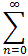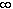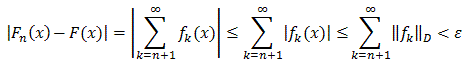#Interactive Real Analysis

Next | Previous | Glossary | Map

## 8.3. Series and Power Series

### Theorem 8.3.3: Weierstrass Convergence Theorem

Suppose fn are a sequence of functions defined on D such that|| fn ||D <where || fn ||D is the sup-norm on D. Then the (function) seriesfn(x) converges absolutely and uniformly on D to a function f. If, in addition, each fn is continuous, the limit function f is also continuous on D.

The proof will involve two steps:

1. we will show that the series converges pointwise to a limit function F
2. the convergence to F is actually uniform

Let's get started with the first step. Fix xD. Then the seriesf(x) is a numeric series. In fact, since |f(x)||| f ||D that numeric series must converge according to the Comparison Test to a limit L. That limit depends on x so that we can a function

F(x) =f(x)

which is well-defined (pointwise) for all xD.

Next we need to show that the convergence is uniform. Take any> 0. Since the numeric series|| fn ||D converges we can find an integer N such that<for all n > N

But then:which means that the sequence of partial sums converges uniformly.

If we knew what uniformly Cauchy meant, we could also show that the sequence of partial sums was uniformly Cauchy, which (we would hope) should imply uniform convergence. To practice, define uniformly Cauchy sequences and finish the proof that way.

If, in addition, each fn is continuous, then every N-partial sum is also continuous. Since uniform convergence preserves continuity, the limit would also be continuous.Next | Previous | Glossary | Map